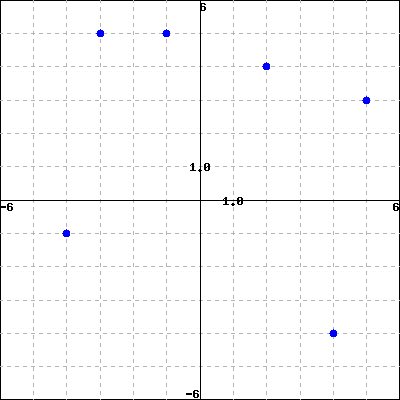This is similar to Problem 6 from your Section 4.1 worksheet.

Consider the function $h$ on the graph below.Find the following.
$h(-1)=$
$h(-3)=$
$h(-4)=$
$h(2)=$